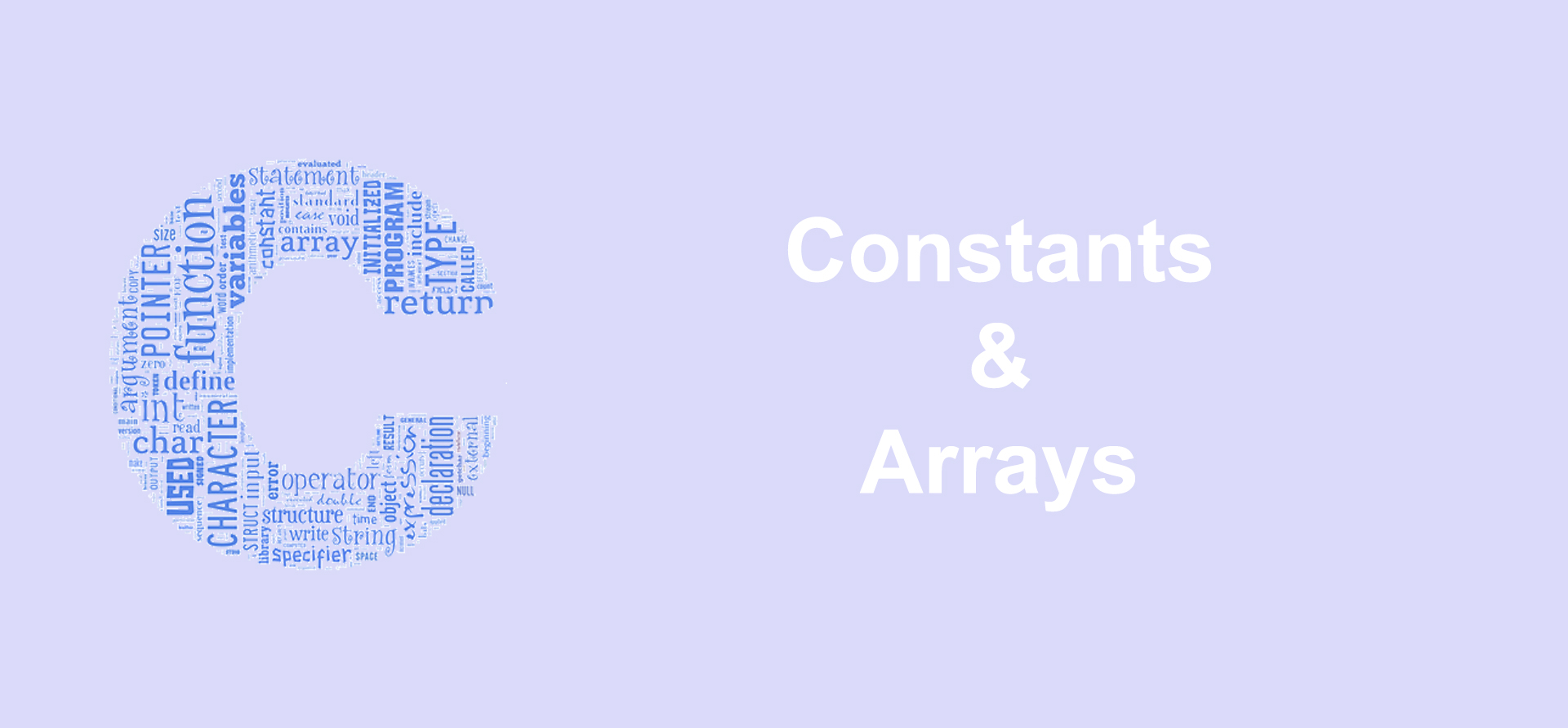Constants and Arrays in C Programming

July 01, 2018 | 1 Tags | 0 CommentConstants

C constants are also like normal variables. But only difference is, their values cannot be modified once they are defined. Constants refer to fixed values. They are also called as literals. If you try to change constant values after defining, it will produce errors.

There are two simple ways in C to define constants

• using #define preprocessor
• using const keyword

By convention, the name of constants are in upper case.

#include <stdio.h>
#include <stdlib.h>

#define PI 3.14159

int main() {
int diameter = 20;

/* calculate square of a circle */
double square = PI * diameter;

printf("Square of the circle is %8.2f\n",square);

/* total value of a product */
const double TAXRATE = 0.1;
double net = 100;

double total = net + net * TAXRATE;

printf("Total = %8.2f\n",total);

return 0;
}
Declaring Arrays

An array is a data structure that stores a collection of values of the same type.

In order to declare an array, you need to specify

• the data type of the array’s elements
• the name of the array
• the number of elements that array may contain

For example, you can declare an array that holds 10 integers as follows:

int a;

When you declare an array, the compiler allocates a memory block that holds the entire array. The array elements are stored sequentially in the memory.

Index of an array starts from 0 to size of array - 1. The first element of a array will be stored at a address and the last element will occupy a.

Initializing Arrays

You can initialize an array in C either one by one

int a;
a = 2;
a = 1;
a = 3;
a = 7;
a = 8;

or using a single statement

int a = {2,1,3,7,8};

The number of values between braces {} cannot be larger than the number of elements between square brackets [].

If you omit the size of the array, the compiler will create an array with the size that is sufficient enough to hold the initialized values.

int a[] = {2,1,3,7,8};

If you initialize just only few array elements, you will not know exact values of the uninitialized elements when the program executes

int a = {2,1,3};

The a and a can hold any integer values.

Accessing Array Elements

You can access an individual element of the array by using the array name followed by the index of the element within square brackets

#include <stdio.h>

int main()
{
const int SIZE = 5;
int a[SIZE];
int i;
for(i = 0; i < SIZE; i++)
{
a[i] = i;
printf("a[%d] = %d\n",i,a[i]);
}
}
Declaring and Initializing Multidimensional Arrays

You can declare a multidimensional array as follows

int matrix;

You can initialize a multidimensional array as follows

int matrix =
{
{11,12,13},
{21,22,23},
{32,31,33},
};

In the following example, first, we declare a two-dimensional array of integers with two rows and three columns. Next we use the scanf() function to read the number from user’s inputs. Then, we display the array content on the screen.

#include <stdio.h>
#include <stdlib.h>

const int ROW = 2;
const int COLUMN = 3;

void fill_array(int (*pm)[COLUMN],int row);
void display(int m[][COLUMN],int row);

int main()
{
int i, j;
int m[ROW][COLUMN];

/* fill array's elements */
fill_array(m, ROW);

/* display array's elements */
display(m, ROW);

return 0;
}

void fill_array(int (*pm)[COLUMN],int row)
{

int i, j;

/* fill array's elements */
for(i = 0; i < row; i++)
{
for(j = 0; j < COLUMN; j++)
{
printf("\nm[%d][%d]:",i,j);
scanf("%d",&pm[i][j]);
}
}
}

void display(int m[][COLUMN],int row)
{
int i, j;
printf("Array's content:\n");

/* display array's elements */
for(i = 0; i < row; i++)
{
for(j = 0; j < COLUMN; j++)
{
printf("%d\t",m[i][j]);
}
printf("\n");
}

}Samuel Yang

If you like this tutorial, you can support me